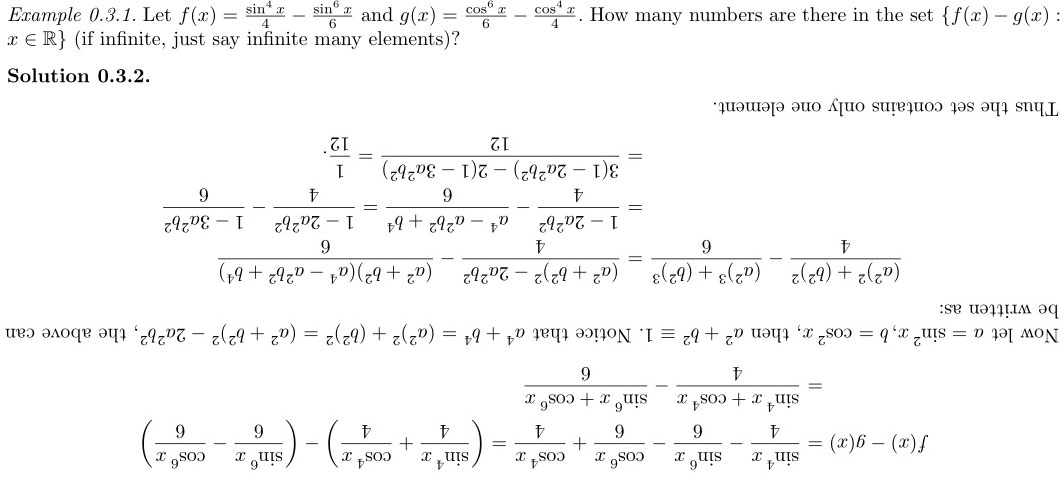## Random posts of math questions and solutions

### Triginometry### Triginometry

Let $$f(x)=\frac{\sin^4 x}{4}-\frac{\sin^6x}{6}$$ and $$g(x)=\frac{\cos^6 x}{6}-\frac{\cos^4x}{4}$$.  How many numbers are there in the set $$\{f(x)-g(x): x\in \mathbb R\}$$ (if infinite, just say infinite many elements)?